Next: Derivation of Snell Resampling Up: Least-squares joint imaging of Previous: Conclusions on the 3-D

# HEMNO Equivalence with Levin and Shah's Equations

In this appendix, I prove that the HEMNO equation is equivalent to Levin and Shah's traveltime equation Levin and Shah (1977) in the limit of small dip angle. They show that in a constant velocity medium with dipping target reflector and multiple generator, the moveout equation of the S102G'' pegleg multiple (see Figure) is:(39)
whereandare the dip angle (in radians) of the multiple generator and target reflector, respectively.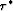andare the zero-offset traveltimes to the two reflectors, x is offset, and V is the medium velocity. For small dip angles (i.e., less than 5 degrees), we can make the small angle approximation for angles,, andto update equation () accordingly: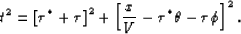(40)
Multiplying out the squares in equation () and collecting terms gives:(41)
Theand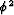terms are negligible for small angles, so we can ignore these terms and further simplify equation ():(42)
I will now show that the HEMNO equation () is equivalent to the Levin/Shah equation () under the constant velocity and small dip angle assumptions. First I make some preliminary definitions. In a constant-velocity medium, the expression for xp, equation (), simplifies to:(43)
Then x-xp, which will be needed later, simplifies to: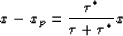(44)
Since the reflectors in this derivation are assumed planar and the velocity is assumed constant, using equations () and (), we can directly write the (two-way) zero offset traveltime to the seabed and subsea reflection at any midpoint as a function of the corresponding zero-offset traveltimes at the midpoint location, y0: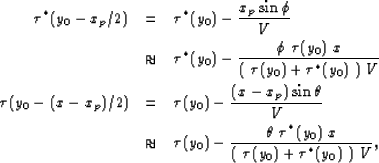(45) (46)
where the small angle approximation was employed as before. Substituting the zero-offset traveltimes () and () into the HEMNO equation () yields: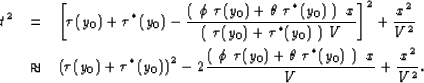(47) (48)
Equation () is equivalent to equation (). Therefore, we have proven the equivalence of the moveout equations of the true and approximate raypaths shown in Figure, subject to the small dip angle approximation. As before,andterms were dropped in going from equation () to equation (). Although explicit seabed and subsea reflector dip angles,and, are contained in equation (), they were introduced only to show equivalence to equation (). Locally-planar reflectors are not required to implement equation ().Next: Derivation of Snell Resampling Up: Least-squares joint imaging of Previous: Conclusions on the 3-D
Stanford Exploration Project
5/30/2004